• 我的框架里面一个多维数组元素操作类，主要用于读取数组中配置数据，可以通过字符串节点的方式：a.b.c 来获取和设置元素，以及多维数组的覆盖，有需求的可以参考下吧！ /** * Created by PhpStorm. * User: ZHOUZ ...
我的框架里面一个多维数组元素操作类，主要用于读取数组中配置数据，可以通过字符串节点的方式：a.b.c 来获取和设置元素，以及多维数组的覆盖，有需求的可以参考下吧！

<?php
/**
* Created by PhpStorm.
* User: ZHOUZ
* Date: 14-5-25
* Time: 下午5:32
*/

namespace Snail\Component;

/**
* 多维数组节点读写
* Class Node
* @package Snail\Component
*/
class Node extends AComponent
{
/**
* 多维数组节点分隔符
* @var string
*/
const SP = '.';

/**
* 将非字符串类型数据生成字符串节点
* <code>
* Node::create(array('a', 'b', 1, true)) => 'a.b.1.1'
* Node::create('a', 'b', null, false)    => 'a.b'
* </code>
* @param mixed $nodes 支持数组、任意参数个数 * @return string */ public static function create($nodes) {
! is_array($nodes) &&$nodes = func_get_args();
return join(static::SP, array_filter(array_map('strval', $nodes))); } /** * 获取多维数组节点转化为数组键名 * @param string$node
* @return array
*/
public static function explode($node) { return explode(static::SP,$node);
}

/**
* 设置多维数组节点值
* 注意：
* 由于 PHP 数组数值键名长度只支持 int 的最大长度，
* 为避免出现覆盖等混乱现象，所有节点在转化为键名时都将
* 强制被转换为字符串类型
* @param array & $arr 引用被操作数组的内存地址 * @param string$node 节点路径, 如: a.b.c => $arr['a']['b']['c']; * @param mixed$value
* @return void
*/
public static function set(array & $arr,$node, $value = null) {$arr = $arr2 = (array)$arr;
$keys = static::explode($node);
foreach ($keys as$key) {
$key = strval($key);
if (isset($arr[$key])) {
$arr = &$arr[$key]; } else {$arr[$key] = array();$arr = & $arr[$key];
}
}
$arr =$value;
}

/**
* 获取多维数组指定节点值
* @param array & $arr 引用被操作数组的内存地址 * @param string$node 节点路径, 如: a.b.c => $arr['a']['b']['c']; * @param null|mixed$default 若节点不存在时返回该默认值
* @return mixed
*/
public static function get(array & $arr,$node, $default = null) { if (empty($arr)) return $default;$keys = static::explode($node); foreach ($keys as $key) {$key = strval($key); if (isset($arr[$key])) {$arr = & $arr[$key];
} else {
return $default; } } return$arr;
}

/**
* 将第二个多维数组覆盖或添加到第一个多维数组进行合并
* @param array & $arraySrc 引用被更新的数组 * @param array &$arrayReplace 引用需要合并的数据
* @return void 地址操作无返回值
*/
public static function merge(array & $arraySrc, array &$arrayReplace) {
if (empty($arrayReplace)) return; foreach ($arrayReplace as $k=>&$v) {
if (is_array($v) && isset($arraySrc[$k])) { static::merge($arraySrc[$k],$v);
} else {
$arraySrc[$k] = $v; } } } /** * 判断多维数组中是否存在指定节点, * 结果同 isset 关键字 (若存在键但值为 NULL 则也会返回 false) * @param array &$arr 引用原数组
* @param string $node * @return bool */ public static function has(array &$arr, $node) { if (empty($arr)) return false;
$keys = static::explode($node);
foreach ($keys as$key) {
$key = strval($key);
if (isset($arr[$key])) {
$arr = &$arr[$key]; } else { return false; } } return true; } /** * 判断多维数组中是否存在指定键 * @param array &$arr 引用原数组
* @param string $node * @return bool */ public static function hasKey(array &$arr, $node) { if (empty($arr)) return false;
$keys = static::explode($node);
foreach ($keys as$key) {
$key = strval($key);
if (array_key_exists($key,$arr)) {
$arr = &$arr[$key]; } else { return false; } } return true; } /** * 注销数组中的指定节点元素 * @param array$arr
* @param string $node * @return void */ public static function clear(array &$arr, $node) { if (empty($arr)) return;
$keys = static::explode($node);
foreach ($keys as$key) {
$key = strval($key);
if (isset($arr[$key])) {
$arr = &$arr[$key]; } else { return; // 指定节点不存在亦认为是成功 } }$arr = null;
unset($arr); } }  下面举个栗子： <?php echo '<pre>'; // 测试数组1$arr1 = ['a'=>['b'=>['c'=>['d'=>['e'=>['f'=>'http://blog.csdn.net/zhouzme']]]]]];
// 测试数组2
$arr2 = ['a'=>['b'=>['c'=>['h'=>['i'=>['j'=>'http://www.hihtml5.com/']]]]]]; echo '读取已存在的多维元素: '; echo Node::get($arr1, 'a.b.c.d.e.f', '默认值');  // http://blog.csdn.net/zhouzme
echo '<hr>';

echo '读取不存在的多维元素: ';
echo Node::get($arr1, 'a.b.c.d.e.g', '默认值'); // 默认值 echo '<hr>'; echo '新增多维元素: '; Node::set($arr1, 'a.b.c.d.e.g', 'http://www.ai9475.com/');
print_r($arr1); echo '<hr>'; echo '读取新增的多维元素: '; echo Node::get($arr1, 'a.b.c.d.e.g', '默认值');  // http://www.ai9475.com/
echo '<hr>';

echo '合并两个数组<br>';
Node::merge($arr1,$arr2);
print_r(\$arr1);

下面是对应的输出结果：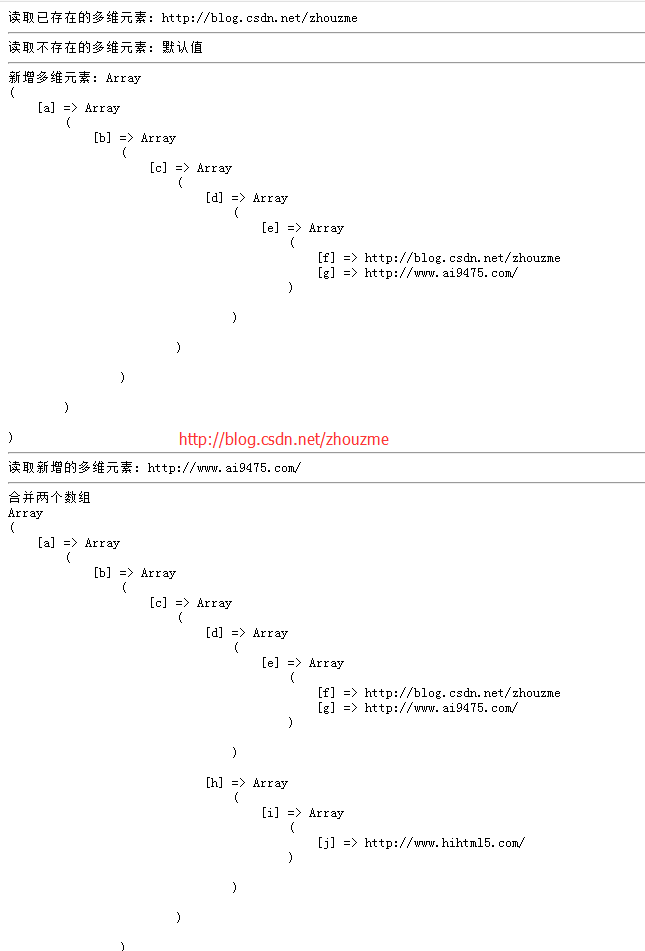展开全文php
• 多维数组元素的地址 转载于:https://www.cnblogs.com/LoveFishC/archive/2012/07/23/3845521.html
多维数组元素的地址
转载于:https://www.cnblogs.com/LoveFishC/archive/2012/07/23/3845521.html
展开全文• 统计多维list中元素出现频率 一、多维list 展开成 一维list 1、定义展开方法 8种方法将list展平为一层(如果是np.array()数据形式直接arr.reshape(-1,1)就OK) import functools import itertools import numpy import...
多维list展开&&统计多维list中元素出现频率
一、多维list 展开成 一维list
1、定义展开方法
8种方法将list展平为一层(如果是np.array()数据形式直接arr.reshape(-1,1)就OK)
import functools
import itertools
import numpy
import operator
import perfplot
from collections import Iterable  # or from collections.abc import Iterable
from iteration_utilities import deepflatten

#使用两次for循环
def forfor(a):
return [item for sublist in a for item in sublist]

#通过sum
def sum_brackets(a):
return sum(a, [])

#使用functools內建模块
def functools_reduce(a):
return functools.reduce(operator.concat, a)

#使用itertools內建模块
def itertools_chain(a):
return list(itertools.chain.from_iterable(a))

#使用numpy
def numpy_flat(a):
return list(numpy.array(a).flat)

#使用numpy
def numpy_concatenate(a):
return list(numpy.concatenate(a))

#自定义函数
def flatten(items):
"""Yield items from any nested iterable; see REF."""
for x in items:
if isinstance(x, Iterable) and not isinstance(x, (str, bytes)):
yield from flatten(x)
else:
yield x

def pylangs_flatten(a):
return list(flatten(a))

#使用库iteration_utilities
def iteration_utilities_deepflatten(a):
return list(deepflatten(a, depth=1))

2、各方法基准时间测试
import matplotlib.pyplot as plt
from simple_benchmark import benchmark

#基准测试
b = benchmark(
[forfor, sum_brackets, functools_reduce, itertools_chain,numpy_flat, numpy_concatenate, pylangs_flatten,iteration_utilities_deepflatten],
arguments={2**i: [*5]*(2**i) for i in range(1, 13)},
argument_name='number of inner lists'
)

#显示测试结果
plt.subplots(1,1,figsize=(15,10))
b.plot()
plt.legend(loc = 'upper left')
plt.show()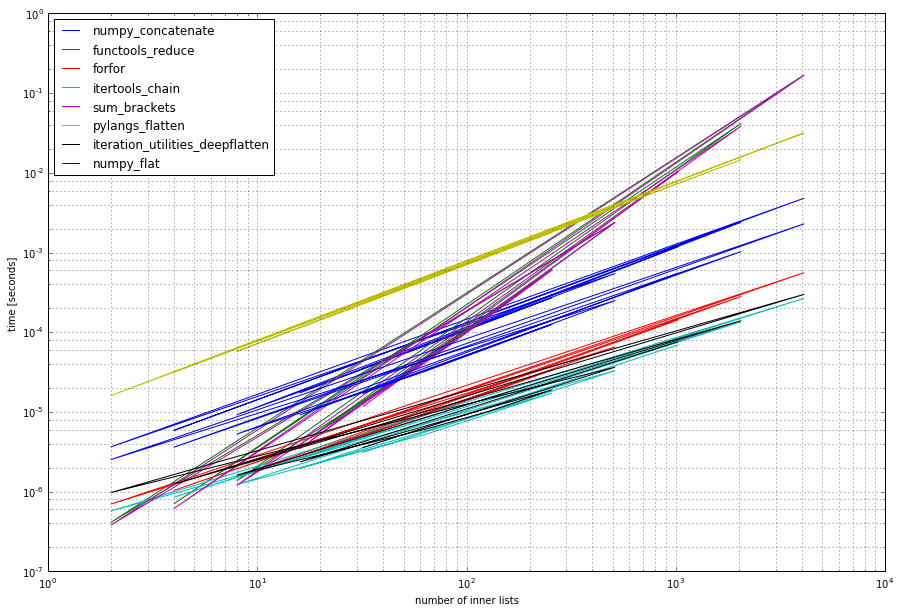参考资料
[Python中多层List展平为一层]
二、统计出现频率
利用Counter 按照降序排好
list1 = [1,2,3,1,1,4]
sorted_x = sorted(Counter(list1.items(), key=lambda x: x, reverse=True)



展开全文• 标准安装的Python中用列表(list)保存一组值，可以用来当作数组使用，不过由于列表的元素可以是任何对象，因此列表中所保存的是对象的指针。这样为了保存一个简单的[1,2,3
http://blog.csdn.net/pipisorry/article/details/39215089

NumPy介绍

Numpy(读作num-pie)是Python中的一个矩阵计算包，功能类似于MATLAB的矩阵计算。

标准安装的Python中用列表(list)保存一组值，可以用来当作数组使用，不过由于列表的元素可以是任何对象，因此列表中所保存的是对象的指针。这样为了保存一个简单的[1,2,3]，需要有3个指针和三个整数对象。对于数值运算来说这种结构显然比较浪费内存和CPU计算时间。

此外Python还提供了一个array模块，array对象和列表不同，它直接保存数值，和C语言的一维数组比较类似。但是由于它不支持多维，也没有各种运算函数，因此也不适合做数值运算。

NumPy的诞生弥补了这些不足，NumPy提供了两种基本的对象：ndarray（N-dimensional array object）和ufunc（universal function object）。ndarray(下文统一称之为数组)是存储单一数据类型的多维数组(同c语言数组直接保存数值，见下面的多维数组ndarray内存结构部分)，而ufunc则是能够对数组进行处理的函数。from:张若愚的《Python科学计算》

具体参见http://www.numpy.org/

安装numpy参见linux和windows中安装python科学计算环境-pycharm、numpy

在Python中使用help帮助

>>> import numpy

>>> help(numpy.argsort)

Numpy中array和ndarray的区别

What is the difference between ndarray and array in numpy?

np.array is just a convenience function to create an ndarray, it is not a class itself.

You can also create an array using np.ndarray, but it is not the recommended way. From the docstring of np.ndarray:

Arrays should be constructed using array, zeros orempty ... The parameters given here refer to a low-level method (ndarray(...)) for instantiating an array.

where can I find the implementations in the numpy source code?

1 Most of the meat of the implementation is in C code, here in multiarray, but you can start looking at the ndarray interfaces here:

https://github.com/numpy/numpy/blob/master/numpy/core/numeric.py

2 array() is implemented in core/src/multiarray/methods.c in array_getarray()

皮皮blog

NumPy中的数据类型

对于科学计算来说，Python中自带的整型、浮点型和复数类型远远不够，因此NumPy中添加了许多数据类型。

Numpy中基础的数据类型是np.dtype类的对象.

需要指定所用数据类型的场合, 比如新生成数组时, 一般都会有个可选参数叫dtype (注意看上面示例). 这个参数可以接受真正的np.dtype对象, 也可以很聪明地直接接收普通的标量类型, 也可以接收各种类型的字符串表示(注意看下面那个大列表里的字符简称). 其默认值一般都是python原生的那种float型(一般就相当于C里面的double).

类似于其他语言的int, float, double之类的标量类型并不是np.dtype类型对象, 但可以用它来构造np.dtype对象. 比如用Python原生的float型来构造:my_type = np.dtype(float)

numpy缺失值的表示(None, np.NaN, np.NaT, pd.NaT)

NaN: not a number, NaN is the default missing value marker forreasons of computational speed and convenience, we need to be able to easilydetect this value with data of different types: floating point, integer,boolean, and general object.

None: treats None like np.nan. In many cases, however, the Python None will arise and we wish to also consider that “missing” or “null”.

NaT:  Datetimes, For datetime64[ns] types, NaT represents missing values. This is a pseudo-native sentinel value that can be represented by numpy in a singular dtype (datetime64[ns]). pandas objects provide intercompatibility between NaT and NaN.

inf: Prior to version v0.10.0 inf and -inf were also considered to be “null” in computations. This is no longer the case by default; use the mode.use_inf_as_null option to recover it.

Note: numpy缺失值的判断要用np.isnan(),而不能使用a == np.NaN.[numpy教程：逻辑函数Logic functions ]

numpy数据类型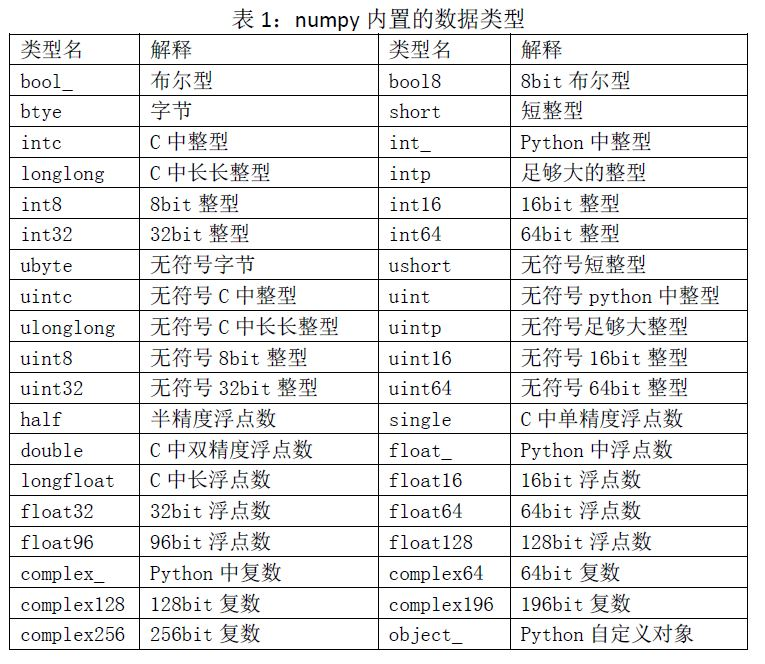Numpy中提供了更细致的标量类型, 见下表,这些基础的标量类型不是np.dtype对象.

每一个numpy内置的数据类型都有一个特征码，它能唯一标识数据类型：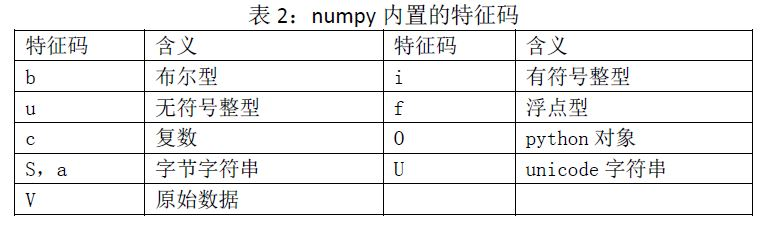采用特征码来表示数据类型时，特征码来表示数据类型时，第一个字符指明数据的种类，其余字符指明每一种数据类型的字节（ 对于字符串来说，该指明字符中字符数）。其中数据 类型的字节数必须与某一个 numpy内置的类型相匹配，否则将会抛出异常。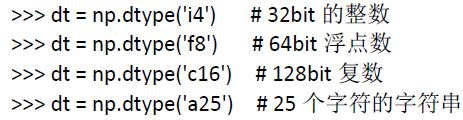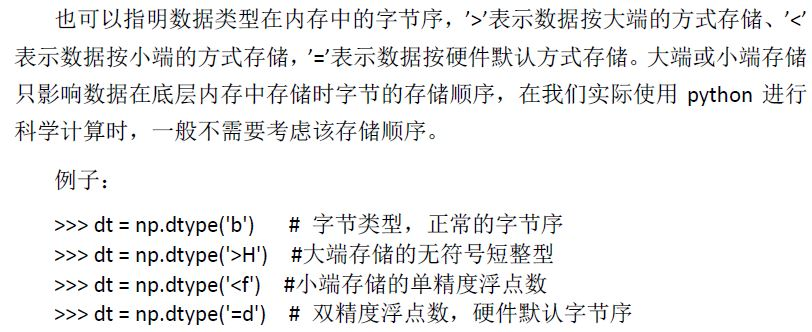np.类型名
对应于别的语言的类型
字符简称
Booleans
np.bool_
Python bool
'?'
np.bool8
8 bit bool

Int
np.byte
C char
'b'
np.short
C short
'h'
np.intc
C int
'i'   #int32
np.int_
Python int
'l'
np.longlong
C long long
'q'
np.intp
用作指针??
'p'
np.int8
np.int16
np.int32
np.int64
特定长度

Unsigned Int
np.ubyte
C unsigned char
'B'
np.ushort
C unsigned short
'H'
np.uintc
C unsigned int
'I'
np.uint_
Python unsigned int
'L'
np.ulonglong
C unsigned long long
'Q'
np.uintp
用作指针??
'P'
np.uint8
np.uint16
np.uint32
np.uint64
特定长度

Float
np.single
C float
'f'
np.double
C double

np.float_
Python float
'd'
np.longfloat
C long float
'g'
np.float32
np.float64
np.float96
np.float128
特定长度
后两个依赖于平台

Complex(复数)
np.csingle

'F'
np.complex_
Python complex
'D'
np.clongfloat

'G'
np.complex64
np.complex128
np.complex192
np.complex256
特定长度
后两个依赖于平台

Any Python Object
np.object_
保存的实际是引用
'O'
np.str_
Python str
'S#' # = number #控制每个str长度
np.unicode_
Python unicode
'U#'
void

'V#'
NumPy中的基本数据类型取值范围

名称
描述
bool
用一个Bit存储的布尔类型（True或False）
inti
由所在平台决定其大小的整数（一般为int32或int64）
int8
一个字节大小，-128 至 127
int16
整数，-32768 至 32767
int32
整数，-2 ** 31 至 2 ** 32 -1
int64
整数，-2 ** 63 至 2 ** 63 - 1
uint8
无符号整数，0 至 255
uint16
无符号整数，0 至 65535
uint32
无符号整数，0 至 2 ** 32 - 1
uint64
无符号整数，0 至 2 ** 64 - 1
float16
半精度浮点数：16位，正负号1位，指数5位，精度10位
float32
单精度浮点数：32位，正负号1位，指数8位，精度23位
float64或float
双精度浮点数：64位，正负号1位，指数11位，精度52位
complex64
复数，分别用两个32位浮点数表示实部和虚部
complex128或complex
复数，分别用两个64位浮点数表示实部和虚部
[NumPy数组（数组初探）]

[Data type objects]

[Data type objects (dtype)]

多维数组ndarray内存结构

下面让我们来看看ndarray数组对象是如何在内存中储存的。如下图所示，关于数组的描述信息保存在一个数据结构中，这个结构引用两个对象：一块用于保存数据的存储区域和一个用于描述元素类型的dtype对象。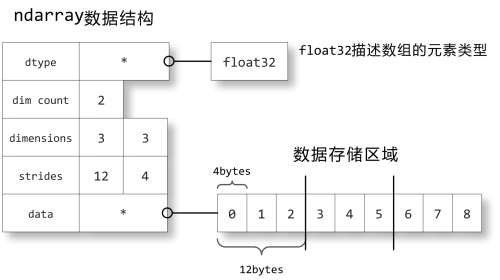ndarray数组对象在内存中的储存方式

数据存储区域保存着数组中所有元素的二进制数据，dtype对象则知道如何将元素的二进制数据转换为可用的值。数组的维数、大小等信息都保存在ndarray数组对象的数据结构中。图中显示的是如下数组的内存结构：

>>> a = np.array([[0,1,2],[3,4,5],[6,7,8]], dtype=np.float32)
a.strides
(12, 4) 

strides中保存的是当每个轴的下标增加1时，数据存储区中的指针所增加的字节数。例如图中的strides为12,4，即第0轴的下标增加1时，数据的地址增加12个字节：即a[1,0]的地址比a[0,0]的地址要高12个字节，正好是3个单精度浮点数的总字节数；第1轴下标增加1时，数据的地址增加4个字节，正好是单精度浮点数的字节数。

如果strides中的数值正好和对应轴所占据的字节数相同的话，那么数据在内存中是连续存储的。然而数据并不一定都是连续储存的，前面介绍过通过下标范围得到新的数组是原始数组的视图，即它和原始视图共享数据存储区域：

>>> b = a[::2,::2]
>>> b
array([[ 0.,  2.],
[ 6.,  8.]], dtype=float32)
>>> b.strides
(24, 8)


由于数组b和数组a共享数据存储区，而b中的第0轴和第1轴都是数组a中隔一个元素取一个，因此数组b的strides变成了24,8，正好都是数组a的两倍。 对照前面的图很容易看出数据0和2的地址相差8个字节，而0和6的地址相差24个字节。

元素在数据存储区中的排列格式有两种：C语言格式和Fortan语言格式。在C语言中，多维数组的第0轴是最上位的，即第0轴的下标增加1时，元素的地址增加的字节数最多；而Fortan语言的多维数组的第0轴是最下位的，即第0轴的下标增加1时，地址只增加一个元素的字节数。在NumPy中，元素在内存中的排列缺省是以C语言格式存储的，如果你希望改为Fortan格式的话，只需要给数组传递order="F"参数：

>>> c = np.array([[0,1,2],[3,4,5],[6,7,8]], dtype=np.float32, order="F")
>>> c.strides
(4, 12)


皮皮blog

ndarray数组维度-横向量和列向量

{关于numpy数组维度表示上的区别，numpy多维数组横向量和列向量的区别}

zeros([5,])    #返回的是一维列向量

[ 0.  0.  0.  0.  0.] #等价于这样的矩阵（一维数组），zeros()、zeros(5)以及zeros(5,)

zeros([5, 1]) #返回的是二维列向量 [[ 0.]  [ 0.]  [ 0.]  [ 0.]  [ 0.]]

zeros([1, 5])    #二维横向量

[[ 0.  0.  0.  0.  0.]]

a = array([1,2,3])    #一维列向量
print(a.shape, a)
b = array([[1,2,3]])    #二维横向量
print(b.shape, b)

(3,) [1 2 3]
(1, 3) [[1 2 3]]


Note:a=np.array([1,2,3]) 由 a.shape知道a是一个列向量，而b=np.array([[1,2,3]])是一个横向量。

b=arange(1,5)   #列向量
print(b.shape, b)

a=array([[1,2,3,4]])#横向量
print(a.shape, a)
print(a+b)

(4,) [1 2 3 4]
(1, 4) [[1 2 3 4]]
[[2 4 6 8]]

Note:

1. 上面的例子没有说明的，数值就是原来的，没有变。比较上面知道，基本上横向量和列向量是可以任意加减的。但是其他的要有一样的shape才可以。

2.一维列向量的转置还是本身。

3. 一维列向量（如(3,)）在广播运算中是当做二维行向量（如(1,3)）计算的。也就是说(3,)相当于(1,3)。

np.mean(二维向量)

返回的是一个一维列向量

numpy.mean的维度：规范化，x矩阵中xi总是以列向量表示一个数据点，行代表维度（行0代表维度1）

A = [[-2. -1.  0.  1.  2.]  [-1.  0. -1.  2.  0.]  [ 1.  1.  1. -1. -2.]]

col_mean = np.mean(A, 0)

print(col_mean.shape)(5,)
[[-1.33333333 -1.          0.          0.33333333  2.        ]
[-0.33333333  0.         -1.          1.33333333  0.        ]

[ 1.66666667  1.          1.         -1.66666667 -2.        ]]

col_mean = np.mean(A, 0).reshape(1, len(A))
print(col_mean.shape)
# print(col_mean)
A -= col_mean
print(A)

(1, 5)
[[-1.33333333 -1.          0.          0.33333333  2.        ]
[-0.33333333  0.         -1.          1.33333333  0.        ]
[ 1.66666667  1.          1.         -1.66666667 -2.        ]]

皮皮blog

array元素存取

元素存取的不同方式

下标范围存取元素

结束索引在这儿是不被包含的！和python中的list的索引相同！但是不同于pandas中的索引是被包含的！！！

数组元素的存取方法和Python的标准方法相同：

>>> a = np.arange(10)
>>> a    # 用整数作为下标可以获取数组中的某个元素
5
>>> a[3:5]  # 用范围作为下标获取数组的一个切片，包括a不包括a
array([3, 4])
>>> a[:5]   # 省略开始下标，表示从a开始
array([0, 1, 2, 3, 4])
>>> a[:-1]  # 下标可以使用负数，表示从数组后往前数
array([0, 1, 2, 3, 4, 5, 6, 7, 8])
>>> a[2:4] = 100,101    # 下标还可以用来修改元素的值
>>> a
array([  0,   1, 100, 101,   4,   5,   6,   7,   8,   9])
>>> a[1:-1:2]   # 范围中的第三个参数表示步长，2表示隔一个元素取一个元素
array([  1, 101,   5,   7])
>>> a[::-1] # 省略范围的开始下标和结束下标，步长为-1，整个数组头尾颠倒
array([  9,   8,   7,   6,   5,   4, 101, 100,   1,   0])
>>> a[5:1:-2] # 步长为负数时，开始下标必须大于结束下标
array([  5, 101])


numpy数组下标是可以越界的！

>>> b
array([1, 2, 3, 4, 5, 6])
>>> b[1:30309]
array([2, 3, 4, 5, 6])
如果越界了，会自动检测，只返回到结尾的数据。

和Python的列表序列不同，通过下标范围获取的新的数组是原始数组的一个视图，它与原始数组共享同一块数据空间。

>>> b = a[3:7] # 通过下标范围产生一个新的数组b，b和a共享同一块数据空间
>>> b
array([101,   4,   5,   6])
>>> b = -10 # 将b的第2个元素修改为-10
>>> b
array([101,   4, -10,   6])
>>> a # a的第5个元素也被修改为10
array([  0,   1, 100, 101,   4, -10,   6,   7,   8,   9])


除了使用下标范围存取元素之外，NumPy还提供了两种存取元素的高级方法。

使用整数序列

当使用整数序列对数组元素进行存取时，将使用整数序列中的每个元素作为下标，整数序列可以是列表或者数组。使用整数序列作为下标获得的数组不和原始数组共享数据空间。

>>> x = np.arange(10,1,-1)
>>> x
array([10,  9,  8,  7,  6,  5,  4,  3,  2])
>>> x[[3, 3, 1, 8]] # 获取x中的下标为3, 3, 1, 8的4个元素，组成一个新的数组；python自带的list不能这么做
array([7, 7, 9, 2])
>>> b = x[np.array([3,3,-3,8])]  #下标可以是负数
>>> b = 100
>>> b
array([7, 7, 100, 2])
>>> x   # 由于b和x不共享数据空间，因此x中的值并没有改变
array([10,  9,  8,  7,  6,  5,  4,  3,  2])
>>> x[[3,5,1]] = -1, -2, -3 # 整数序列下标也可以用来修改元素的值
>>> x
array([10, -3,  8, -1,  6, -2,  4,  3,  2])


使用布尔数组

当使用布尔数组b作为下标存取数组x中的元素时，将收集数组x中所有在数组b中对应下标为True的元素，使用布尔数组作为下标获得的数组不和原始数组共享数据空间。

注意这种方式只对应于布尔数组（np.array([True, False, ....])），不能使用布尔列表([True, False, ....])。

使用布尔数组进行numpy数组值的替换：a[ a == 3] = 4; ll_array[np.array(labels_list) == label] = km_model.centers[label]

>>> x = np.arange(5,0,-1)
>>> x
array([5, 4, 3, 2, 1])
>>> x[np.array([True, False, True, False, False])]
>>> # 布尔数组中下标为0，2的元素为True，因此获取x中下标为0,2的元素
array([5, 3])
>>> x[[True, False, True, False, False]]
>>> # 如果是布尔列表，则把True当作1, False当作0，按照整数序列方式获取x中的元素
array([4, 5, 4, 5, 5])
>>> x[np.array([True, False, True, True])]
>>> # 布尔数组的长度不够时，不够的部分都当作False
array([5, 3, 2])
>>> x[np.array([True, False, True, True])] = -1, -2, -3
>>> # 布尔数组下标也可以用来修改元素
>>> x
array([-1,  4, -2, -3,  1])


布尔数组一般不是手工产生，而是使用布尔运算的ufunc函数产生，关于ufunc函数请参照 ufunc运算 一节。

>>> x
array([ 0.72223939,  0.921226  ,  0.7770805 ,  0.2055047 ,  0.17567449,
0.95799412,  0.12015178,  0.7627083 ,  0.43260184,  0.91379859])
>>> x[x>0.5]
array([ 0.72223939,  0.921226  ,  0.7770805 ,  0.95799412,  0.7627083 , 0.91379859])


多维数组切片操作

多维数组的存取和一维数组类似，因为多维数组有多个轴，因此它的下标需要用多个值来表示，NumPy采用组元(tuple)作为数组的下标。如下图所示，a为一个6x6的数组，图中用颜色区分了各个下标以及其对应的选择区域。

虽然我们经常在Python中用圆括号将组元括起来，但是其实组元的语法定义只需要用逗号隔开即可,不需要圆括号，例如 x,y=y,x 就是用组元交换变量值的一个例子。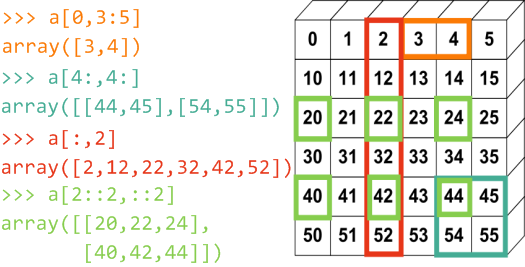使用数组切片语法访问多维数组中的元素

如何创建这个数组: 数组a实际上是一个加法表，纵轴的值为0, 10, 20, 30, 40, 50；横轴的值为0, 1, 2, 3, 4, 5。纵轴的每个元素都和横轴的每个元素求和，就得到图中所示的数组a。你可以用下面的语句创建它，至于其原理我们将在后面的章节进行讨论：

>>> np.arange(0, 60, 10).reshape(-1, 1) + np.arange(0, 6)
array([[ 0,  1,  2,  3,  4,  5],
[10, 11, 12, 13, 14, 15],
[20, 21, 22, 23, 24, 25],
[30, 31, 32, 33, 34, 35],
[40, 41, 42, 43, 44, 45],
[50, 51, 52, 53, 54, 55]])


多维数组同样也可以使用整数序列和布尔数组进行存取

假设x是一个二维数组，inds是整数序列的数组array类型表示的下标：

如果inds是一个一维array，则x[inds]返回的是一个二维array；但是如果inds是一个二维array，则x[inds]返回的是一个三维array。

import numpy as np

x = np.array([[1, 2, 3], [2, 3, 4]])
inds = np.array([0, 1])
print(x[inds].shape, '\n', x[inds])
inds = np.array([[0, 1]])
print(x[inds].shape, '\n', x[inds])

(2, 3)
[[1 2 3]
[2 3 4]]
(1, 2, 3)
[[[1 2 3]
[2 3 4]]]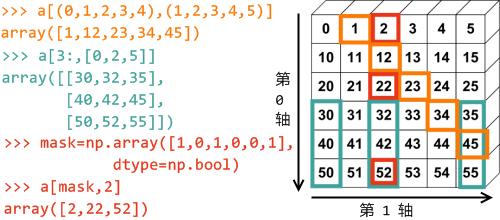使用整数序列和布尔数组访问多维数组中的元素

a[(0,1,2,3,4),(1,2,3,4,5)] : 用于存取数组的下标和仍然是一个有两个元素的组元，组元中的每个元素都是整数序列，分别对应数组的第0轴和第1轴。从两个序列的对应位置取出两个整数组成下标： a[0,1], a[1,2], ..., a[4,5]。

a[3:, [0, 2, 5]] : 下标中的第0轴是一个范围，它选取第3行之后的所有行；第1轴是整数序列，它选取第0, 2, 5三列。

结构数组

在C语言中我们可以通过struct关键字定义结构类型，结构中的字段占据连续的内存空间，每个结构体占用的内存大小都相同，因此可以很容易地定义结构数组。和C语言一样，在NumPy中也很容易对这种结构数组进行操作。只要NumPy中的结构定义和C语言中的定义相同，NumPy就可以很方便地读取C语言的结构数组的二进制数据，转换为NumPy的结构数组。

假设我们需要定义一个结构数组，它的每个元素都有name, age和weight字段。在NumPy中可以如下定义：

import numpy as np
persontype = np.dtype({
'names':['name', 'age', 'weight'],
'formats':['S32','i', 'f']})
a = np.array([("Zhang",32,75.5),("Wang",24,65.2)],
dtype=persontype)


我们先创建一个dtype对象persontype，通过其字典参数描述结构类型的各个字段。字典有两个关键字：names，formats。每个关键字对应的值都是一个列表。names定义结构中的每个字段名，而formats则定义每个字段的类型：

S32 : 32个字节的字符串类型，由于结构中的每个元素的大小必须固定，因此需要指定字符串的长度

i : 32bit的整数类型，相当于np.int32

f : 32bit的单精度浮点数类型，相当于np.float32

然后我们调用array函数创建数组，通过关键字参数 dtype=persontype， 指定所创建的数组的元素类型为结构persontype。运行上面程序之后，我们可以在IPython中执行如下的语句查看数组a的元素类型

>>> a.dtype
dtype([('name', '|S32'), ('age', '<i4'), ('weight', '<f4')])


这里我们看到了另外一种描述结构类型的方法： 一个包含多个组元的列表，其中形如 (字段名, 类型描述) 的组元描述了结构中的每个字段。类型描述前面为我们添加了 "|", "<" 等字符，这些字符用来描述字段值的字节顺序：

| : 忽视字节顺序

< : 低位字节在前

> : 高位字节在前

结构数组的存取方式和一般数组相同，通过下标能够取得其中的元素，注意元素的值看上去像是组元，实际上它是一个结构：

>>> a
('Zhang', 32, 75.5)
>>> a.dtype
dtype([('name', '|S32'), ('age', '<i4'), ('weight', '<f4')])


a是一个结构元素，它和数组a共享内存数据，因此可以通过修改它的字段，改变原始数组中的对应字段：

>>> c = a
>>> c["name"] = "Li"
>>> a["name"]
"Li"


结构像字典一样可以通过字符串下标获取其对应的字段值：

>>> a["name"]
'Zhang'


我们不但可以获得结构元素的某个字段，还可以直接获得结构数组的字段，它返回的是原始数组的视图，因此可以通过修改b改变a["age"]：

>>> b=a[:]["age"] # 或者a["age"]
>>> b
array([32, 24])
>>> b = 40
>>> a["age"]
40


通过调用a.tostring或者a.tofile方法，可以直接输出数组a的二进制形式：

>>> a.tofile("test.bin")


利用下面的C语言程序可以将test.bin文件中的数据读取出来。

内存对齐

C语言的结构体为了内存寻址方便，会自动的添加一些填充用的字节，这叫做内存对齐。例如如果把下面的name改为name的话，由于内存对齐问题，在name和age中间会填补两个字节，最终的结构体大小不会改变。因此如果numpy中的所配置的内存大小不符合C语言的对齐规范的话，将会出现数据错位。为了解决这个问题，在创建dtype对象时，可以传递参数align=True，这样numpy的结构数组的内存对齐和C语言的结构体就一致了。

#include <stdio.h>

struct person
{
char name;
int age;
float weight;
};

struct person p;

void main ()
{
FILE *fp;
int i;
fp=fopen("test.bin","rb");
fclose(fp);
for(i=0;i<2;i++)
printf("%s %d %f", p[i].name, p[i].age, p[i].weight);
getchar();
}


结构类型中可以包括其它的结构类型，下面的语句创建一个有一个字段f1的结构，f1的值是另外一个结构，它有字段f2，其类型为16bit整数。

>>> np.dtype([('f1', [('f2', np.int16)])])
dtype([('f1', [('f2', '<i2')])])


当某个字段类型为数组时，用组元的第三个参数表示，下面描述的f1字段是一个shape为(2,3)的双精度浮点数组:

>>> np.dtype([('f0', 'i4'), ('f1', 'f8', (2, 3))])
dtype([('f0', '<i4'), ('f1', '<f8', (2, 3))])


用下面的字典参数也可以定义结构类型，字典的关键字为结构中字段名，值为字段的类型描述，但是由于字典的关键字是没有顺序的，因此字段的顺序需要在类型描述中给出，类型描述是一个组元，它的第二个值给出字段的字节为单位的偏移量，例如age字段的偏移量为25个字节：

>>> np.dtype({'surname':('S25',0),'age':(np.uint8,25)})
dtype([('surname', '|S25'), ('age', '|u1')])

[http://mp.weixin.qq.com/s?__biz=MjM5NzU0MzU0Nw==&mid=204507794&idx=2&sn=56f4950a7399af1b1bf69e194d55a48a&scene=1&from=singlemessage&isappinstalled=0#rd]

掩码数组

{表示的是不完整的数据或是含有无效值的数据}

>>> import numpy.ma as ma
>>> b
array([2, 3, 3, 0, 1, 4, 2, 4])
>>> mx
masked_array(data = [-- 3 3 -- -- 4 -- 4],
mask = [ True False False  True  True False  True False],fill_value = 999999)

接下来就可以对这些数据操作了

点(…)

代表许多产生一个完整的索引元组必要的分号。如果x是秩为5的数组(即它有5个轴)，那么:

x[1,2,…] 等同于 x[1,2,:,:,:],
x[…,3] 等同于 x[:,:,:,:,3]
x[4,…,5,:] 等同 x[4,:,:,5,:].
>>> c = array( [ [[  0,  1,  2],      # a 3D array (two stacked 2D arrays)
...               [ 10, 12, 13]],
...
...              [[100,101,102],
...               [110,112,113]] ] )
>>> c.shape
(2, 2, 3)
>>> c[1,...]                          # same as c[1,:,:] or c
array([[100, 101, 102],
[110, 112, 113]])
>>> c[...,2]                          # same as c[:,:,2]
array([[  2,  13],
[102, 113]])
from:http://blog.csdn.net/pipisorry/article/details/39215089

ref: NumPy Reference*

中文 Python 笔记：03.numpy*

《Python科学计算》
《Tentative NumPy Tutorial》

100 numpy exercises


展开全文numpy 基本数据类型 数据存储
• 今天小编就为大家分享一篇python实现在多维数组中挑选符合条件的全部元素，具有很好的参考价值，希望对大家有所帮助。一起跟随小编过来看看吧...